### Scribe Post for December 08, 2009

Tuesday, December 8, 2009

Ehy guys!
Today in class we did a Math test about adding and subtracting fractions and for that there is not to much to say..
I believe that almost everyone has done a good job in the test!
Anyway after the test Mr Backey has given to all "some" math sheets(fractions review)to do for homework(for tomorrow the first one)!
So do not forget to do the homework!
sorry if im late
or for some errors :P
in the next scribe ill pick Mark :D
dont forget to leave a comment :-)
see ya guys!

### Mathematics Scribe post for December 4, 2009

Sunday, December 6, 2009

Renz Adame's Blog postHello everybody, this is Renz A. and this is the blogpost for Friday, December 4th, 2009.

Today we worked on our unfinished homework on Practice page 68 of the text book the whole class. We didn't do much and i cant really blog much about it except that i can put up pictures to show how to do some of the questions.

Some of the questions are:

Page 68:

#5. a)
(Click on the page to enlarge)#6. a)These are just some of the questions from the homework and there is more but this is all for now.

The next scribe is..............................................................Giulio

### Scribe Post for December 03, 2009

Thursday, December 3, 2009

Hey everyone.

Yesterday, no one post the scribe, so lets talked a little bit about that :).

• So the things we did yesterday was to write the "Keyideas" on page 67 in our own words.
• And we also answered the "Communicate the Ideas" on page 67 and 68.
Now, lets talked about the things we did today.

Sign(negative,positive) of Multiplying and Dividing
-When multiplying and dividing integers(negative and positive), if there is an odd number of negative signs then the answer will be negative.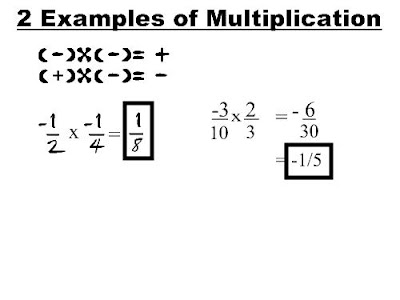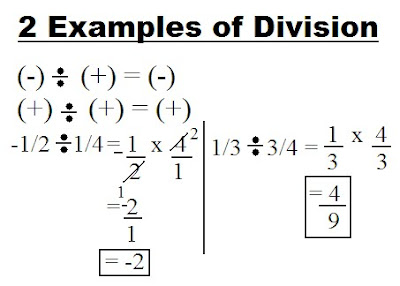And then we answered our homework...
Communicate the Ideas [pg. 67 and 68] Here are the answers:

1]• c.) The common denominator I prefer is 12 because its easier to simplify and because the "MATHEMATICIANS ARE LAZY!".
2]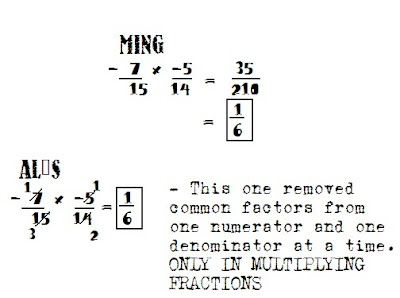3]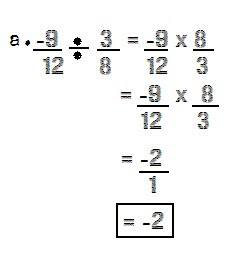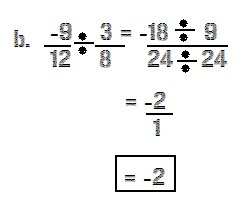• c.) A. Because its easier.
4]
• Because sometimes you forget to put the sign after you solved it.

_______________________________________________________

9-06 Dont Forget to do our homework on the book.
Heres the online link for the ebook "CLICKY HERE!".
The homework is at "PRACTISE ON PAGE 68-69".

For the next scribe I pick.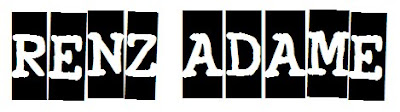hahah *peace*...

SORRY FOR ALL THE MISTAKES :)

Dont FORGET TO Drop some COMMENT[s].

Uhm Mr. B Sorry If i posted it late because I forgot that im doing the scribe *sorry*.

### FRACTIONS Countinues..

Friday, November 27, 2009

On thursday for math we did two different things. First we did some multiplying and dividing fractions which is new.

We did some easy questions like.

and we did another one with DECIMAL

Just so everyone knows when you see a big black dot like this...

Anyways we did someother things like this.. and we learned about RECIPROCAL.

These are all called the

and that mean

So in order to do this we have to find the reciprocal

And now i'm going to talk about DIVIDING Fractions.

After we find the Reciprocal we have to multiply the question then wil get the answer.

Then we divide the reciprocal by 1.

Then we have to multiply it so we can get the answer.

And that is how you do DIVIDING FRACTIONS.
That's it for TODAY.. srry it's late..
anyways the next scribe is
LARA.. GOOD LUCK !!

PLEASE COMMENT!!!

IF YOU NEED HELP WITH ANY OF THIS. GO TO MATH HELP AT LUNCH TIME. MATH HELP IS GOING TO MAKE YOUR LIFE VERY EASY.

Tuesday, November 24, 2009

Today In Math Class We Learned , When Adding and subtracting fractions , To do so you have to find a common denominator If Necessary . Add And subtract Numerators denominators Have to be The Same The Simplify .

This Image Shows Adding Fractions . It Is also The same When You Subtract But The numerator numbers wil be subtrac
ted insteadWe Also Learned In class how to Multipy Fractions .
When We Multiply Fractions , Simply multiply The Numerators Together and then multiply the Denominaters Together , Then Simplify If Possible :)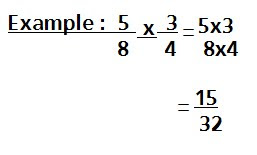For HomeWork

We Had 10 questions to do :)

1 : 2/3 x 3/5 =
2 : 4/5 x 2/9 =
3 : 7/8 x 4/5 =
4 : 9/10 x 5/6 =
5 : 2/5 x 3/4 =
6 : 1/9 x 1/9 =
7 : -1/2 x 2/3 =
8 : -2/7 x 7/2 =
9 : -4/5 x -5/4 =
10: 6/7 x 1 1/6 =

And also Page 2.2 in Our math books :)
I choose Raye To do the next Scribe :)### Scribe Post

Today in class we went into the computer lab and we went on a site and played a game with Adding and Subtracting Mixed numbers..
This is an example for the adding mixed number and subtracting mixed numbers.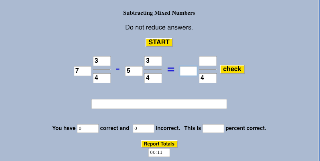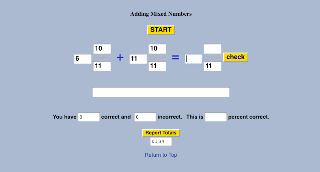### Scribe Post for November 20th

Friday, November 20, 2009

Hey guys! (: On Friday in math class we learned about more fractions. We also went over the homework from Thursday.

Here's a question that some people had troubles with from the homework on Thursday: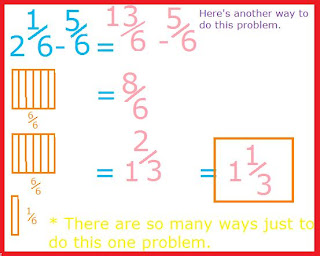Now here's a question Mr. Backé asked us to try in class on Friday:

*Mr Backé showed us 2 different ways to solve this.

Here's one way:

Here's a second way:

HOMEWORK!

1) 2 7/8 - 1 1/4=

2) 3 5/6 - 2 2/3=

3) 11 3/4 - 2 7/8=

4) 5 1/2 - 5/6=

5) 3 1/8 - 1 1/6=

I pick Nicole N for the next scribe. =)

### Scribe Post for November 19th.

Thursday, November 19, 2009

Hey Guys. (: Today in class we just went over our homework, and practiced some questions that people had some trouble with.

At first, we went over a question that people had some trouble with on homework. This was the question:This is how to figure it out.

1st Step -
Rewrite the question and solve the symbols (-,+).

2nd Step -
Figure out the common denominators.Our homework for today:

1) 5 - 2/3

2) 4 - 5/6

3) 10 - 7/8

4) 5 - 2 2/3

5 6 - (-2/5)

I pick Courtney for the next scribe. :) Oh, and comment like crazy guys. x)

### FRACTIONS

Wednesday, November 18, 2009

HELLO PEOPLE FROM 9-06! Today for the SCRIBE POST I'm going to talk about some questions from yesterday and today. AND SORRY for any mistakes!!

So lets begin!!!
This is one of the QUESTIONS we did.
Usually we would find COMMON DENOMINATOR but for this question we don't have to because they are both the same.
Anyways after we have to find the EQUIVALENT FRACTIONS. We don't have to find the equivalent fraction for this one because we didn't have to find the denominators.

Then we have to REWRITE THE QUESTION . Then we answer the question.That was an example of ADDING NEGATIVE FRACETIONS.

These here are very IMPORTANT.

(-)=(-)

-(-)=(+)

-(-)(-)=(-)

-(-)(-)(-)=(+)

-(2/3)=-2/3

-(-2/3)=2/3

-(-2/-3)=-2/-3

Now i'm going to give examples of SUBTRACTING NEGATIVE FRACTIONS

The first thing you have to do is... FIND COMMON DENOMINATORS and it seem like this one already has commonSo.. For this one we don't have to find the EQUIVALENT FRACTION. So will go to the next step which is REWRITE AND ANSWER.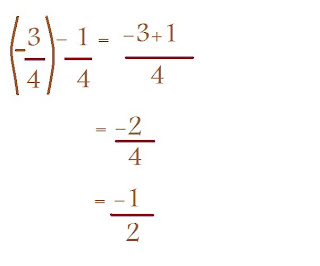PLEASE LEAVE A COMMENT!!!!
AND THE NEXT SCRIBE IS
RAYE FLETT..
THAT IS IF YOU DID NOT DO ONE

SEE YAAHH NEXT TIME :P

### Scribe post for November 17 2009

Tuesday, November 17, 2009

Tuesday, November 17 ,2009
What's up guys! today we talked about fractions and as always we first clarified and corrected homework for today.
the number ten was the one that seemed more difficult, but here is the solution for you =)
:First we need to find the denominator....and that is 60!
and as second we need to calculate the numerator and that will be:77/60=17/60 and thats the answer!
anyway after that we did exercises on fractions like that:and that:.....that's it!
Hope that you understood how to proper fractions(in my first subscribe xP)!

thanks for
Jericho to choose me ;)
anyway don't forget to leave a comment and the homework for tomorrow!!
see ya!

### Scribe Post for November 16, 2009

Monday, November 16, 2009

Hey guys today in class we talked about adding proper fractions. So how do we add proper fractions? I'll show you how step by step.

3/4 + 1/6

Step 1: Find a common denominator. The common denominator is 12. For those who doesn't know how to find the it, I will show you how.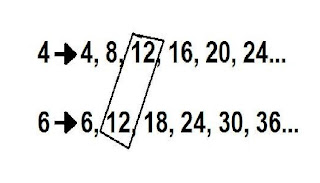Okay, so that is how you can find the common denominator.

Step 2: Find equivalent fractions.Step 3: Rewrite the question. Now you have the same denominator you can answer it.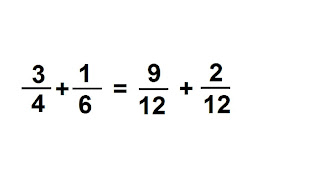Step 4: Answer and simplify if possible.So that's it. The answer is 11/12. It is already simplified. Note: When you add or subtract fractions, you only add or subtract the numerators.

Homework
:
Answer these questions Mr. B gave us:

1) 3/8 + 3/4

2) 1/6 + 1/4

3) 1/5 + 1/3

4) 3/10 + 3/4

5) 1/16 + 1/8

6) 1/2 + 3/5

7) 4/7 + 3/14

8) 3/4 + 7/8

9) 1/9 + 17/18

10) 1/5 + 1/4 + 1/3 +1/2

Okay, if you're having a really bad time with those difficult questions, follow the steps so you can answer those questions easily ;)

### Scribe Post for November 13th, 2009

Saturday, November 14, 2009

Friday ,November 13
Today we went to the computer lab to learn more about fraction at nlvm.usu.edu
First got to Number and Operations (Grade 9-12)and then to Fractions-adding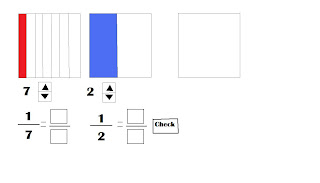This is somehow it is look like. What we need to do here is to find out the sum of the fractions shown. First you should rename the fractions 1/7 and 1/2 so their denominators would be the same.Now the denominators are the same you can now add the fractions.

When adding fractions with different denominators you should rename it with its equivalent fraction to make the denominator the same.

After that we went to lumosity.com, created accounts then played some games about math.

After we leave Mr. Backe told us a homework which Show You Know in chapter 2.3.

### Scribe Post for November 3, 2009

Tuesday, November 3, 2009

Hello everyone.Today in class we talked about Jayden's number line post and we talked about Adding, Subtracting, Multiplying, and Dividing integers.We had to talk about Jayden's number line because the fractions were at the wrong place.

Here is an example of Jayden's picture:Mr.B told us to talk to our tables and see what's wrong with it.I asked Renz P what's wrong with it and he said that -2/9 should be closer to zero and -4/7 should be after -2/9 i asked him why and he said becuase -2/9 is 0.2 and -4/7 is 0.5 so -2/9 should be closer to zero.

Here is an example that Mr.B gave us :Here is the second one: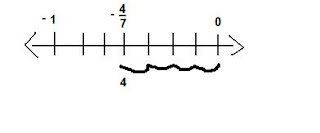He told us to put each fractions in a different number line.

For the rest of the class Mr. B gave us Multiplying and Dividing Integers and told us to give 2 examples for each one.

Multiplying :

(+) x (+) =
(5) x (8) = 40
(7) x (5) = 35
(+) x (-) =
(8) x (-5) = -40
(9) x (-2) = -18
(-) x (+) =
(-6) x (7) = -42
(-4) x (7) = -28
(-) x (-) =
(-8) x (-5) = 40
(-2) x (-4) = 8

Dividing :

(+) ÷ (+) =
(3) ÷ ( 4) = 0.75
(4) ÷ (7) = 0.5
(+) ÷ (-) =
(4) ÷ (-9) = -0.4
(9) ÷ (-7) = -1.2
(-) ÷ (+) =
(-5) ÷ (4) = 1.25
(-9) ÷ (2) = -4.5
(-) ÷ (-) =
(-3) ÷ (-6) = 0.5
(-5) ÷ (-3) = 1.6

Homework for November 3,2009

Page 57,58 and 59 (syk)
and check your understanding (CYU). Then practice page 60 # 4 or 5, and each of # 8, 9, 10 and 11.

Well that's it for me,it took me 2 days to do this please tell me if there's any mistakes,errors or anything at all comment.Thats it for today and now i have to do math homework.BYE!